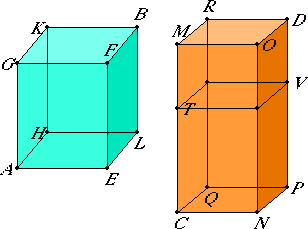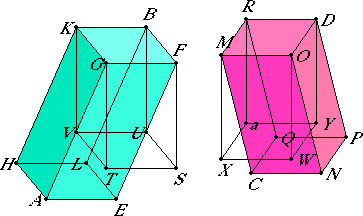# Proposition 34

In equal parallelepipedal solids the bases are reciprocally proportional to the heights; and those parallelepipedal solids in which the bases are reciprocally proportional to the heights are equal.

Let AB and CD be equal parallelepipedal solids.

I say that in the parallelepipedal solids AB and CD the bases are reciprocally proportional to the heights, that is the base EH is to the base NQ as the height of the solid CD is to the height of the solid AB.

First, let the sides which stand up, namely AG, EF, LB, HK, CM, NO, PD, and QR, be at right angles to their bases.

I say that the base EH is to the base NQ as CM is to AG.XI.32

If now the base EH equals the base NQ, and the solid AB equals the solid CD, then CM equal AG. For parallelepipedal solids of the same height are to one another as the bases, and the base EH is to NQ as CM is to AG, and it is clear that in the parallelepipedal solids AB and CD the bases are reciprocally proportional to the heights.

Next, let the base EH not be equal to the base NQ, but let EH be greater.

Now the solid AB equals the solid CD, therefore CM is also greater than AG.

Make CT equal to AG, complete the parallelepipedal solid VC on NQ as base with CT as height.

V.7

Now, since the solid AB equals the solid CD, and CV is outside them, and equals have to the same the same ratio, therefore the solid AB is to the solid CV as the solid CD is to the solid CV.

But the solid AB is to the solid CY as the base EH is to the base NQ, for the solids AB and CV are of equal height, and the solid CD is to the solid CV as the base MQ is to the base TQ and CM is to CT, therefore the base EH is to the base NQ as MC is to CT.

But CT equals AG, therefore the base EH is to the base NQ as MC is to AG.

Therefore in the parallelepipedal solids AB and CD the bases are reciprocally proportional to the heights.

Again, in the parallelepipedal solids AB and CD let the bases be reciprocally proportional to the heights, that is the base EH is to the base NQ, so let the height of the solid CD be to the height of the solid AB.

I say that the solid AB equals the solid CD.

Let the sides which stand up be at right angles to the bases.

Now, if the base EH equals the base NQ, and the base EH is to the base NQ as the height of the solid CD is to the height of the solid AB, therefore the height of the solid CD also equals the height of the solid AB.

But parallelepipedal solids on equal bases and of the same height equal one another, therefore the solid AB equals the solid CD.

XI.31

Next, let the base EH not be equal to the base NQ, but let EH be greater.

Therefore the height of the solid CD is also greater than the height of the solid AB, that is, CM is greater than AG.

Make CT equal to AG again, and complete the solid CV.

Since the base EH is to the base NQ as MC is to AG, and AG equals CT, therefore the base EH is to the base NQ as CM is to CT.

But the base EH is to the base NQ as the solid AB is to the solid CV, for the solids AB and CV are of equal height, and CM is to CT as the base MQ is to the base QT and as the solid CD is to the solid CV.

V.9

Therefore the solid AB is to the solid CV as the solid CD to the solid CV. Therefore each of the solids AB and CD has to CV the same ratio. Therefore the solid AB equals the solid CD.

X.11

Now let the sides which stand up, FE, BL, GA, HK, ON, DP, MC, and RQ, not be at right angles to their bases. Draw perpendiculars from the points F, G, B, K, O, M, D, and R to the planes through EH and NQ, and let them meet the planes at S, T, U, V, W, X, Y, and a. Complete the solids FV and Oa.I say that, in this case too, if the solids AB and CD are equal, then the bases are reciprocally proportional to the heights, that is, the base EH is to the base NQ as the height of the solid CD to the height of the solid AB.

Since the solid AB equals the solid CD, and AB equals BT, for they are on the same base FK and of the same height, and the solid CD equals DX, for they are again on the same base RO and of the same height, therefore the solid BT also equals the solid DX.

Above

Therefore the base FK is to the base OR as the height of the solid DX is to the height of the solid BT. But the base FK equals the base EH, and the base OR equals the base NQ, therefore the base EH is to the base NQ as the height of the solid DX is to the height of the solid BT.

But the solids DX and BT and the solids DC and BA have the same heights respectively, therefore the base EH is to the base NQ as the height of the solid DC is to the height of the solid AB.

Therefore in the parallelepipedal solids AB and CD the bases are reciprocally proportional to the heights.

Next, in the parallelepipedal solids AB and CD let the bases be reciprocally proportional to the heights, that is, as the base EH is to the base NQ, so let the height of the solid CD be to the height of the solid AB.

I say that the solid AB equals the solid CD.

With the same construction, since the base EH is to the base NQ as the height of the solid CD is to the height of the solid AB, and the base EH equals the base FK, and NQ equals OR, therefore the base FK is to the base OR as the height of the solid CD is to the height of the solid AB.

But the solids AB and CD and the solids BT and DX have the same heights respectively, therefore the base FK is to the base OR as the height of the solid DX is to the height of the solid BT.

Above

Therefore in the parallelepipedal solids BT and DX the bases are reciprocally proportional to the heights. Therefore the solid BT equals the solid DX.

But BT equals BA, for they are on the same base FK and of the same height, and the solid DX equals the solid DC. Therefore the solid AB also equals the solid CD.

Therefore, in equal parallelepipedal solids the bases are reciprocally proportional to the heights; and those parallelepipedal solids in which the bases are reciprocally proportional to the heights are equal.

Q.E.D.

## Guide

A proof of this proposition could be made with very little regard to geometry but almost entirely in terms of abstract proportions. The volume of a parallelepiped is proportional to its base for equal heights (XI.32), and proportional to its height for equal bases (not actually stated by Euclid), therefore the base and height are inversely proportional for equal volumes. Such a proof, although simpler, is not in Euclid’s style.

This proposition is used in the proof of proposition XII.9, an analogous statement about pyramids.